# A Guide to Choosing a Proper Inductor for DC-DC Boost Applications

There are numerous DC-DC application areas such as medical (portable), automotive, telecommunication and more. This article will discuss how to choose a proper inductor using step-up DC-DC known as ‘Boost Converter.’

A simplified boost converter circuit can be seen in Figure 1. the input voltage of this converter is always smaller than the output voltage. The boost converter works in the following way; the current flows through inductor L1 and the S1 when S1 is closed, charging L1 but no power will deliver to the load. When S1 is open, the voltage across the load equals the dc input voltage and the charge stored in the L1 is delivering current to the load.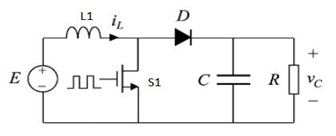Figure 1. Simplified Boost Circuit

You must define or calculate the following parameters when selecting an inductor for a Boost Converter; (the values at right side of the equal signs are given for calculation purpose only). This design example focuses on the “discontinuous mode”.

• Frequency = f = 500 KHz
• V output = 36 V
• I output = 1.5 Amps
• V input nom = 12 V
• V input min = 10.8 V
• V input max = 13.5 V
• Dwell time (dead time) duty ratio = 0.1
• V diode drop = 0.7 V
• R on (FET) = 50 mΩ

## Calculations

Since the operating frequency is 500 KHz,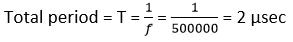Find the output power, P out

P out = (V out + V diode drop) (I output)

P out = (36 + 0.7) (1.5) = 55 W

Find the maximum current input, I input max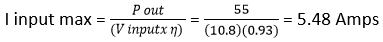Find the FET volt drop, V fet volt drop

V fet volt drop = I in (max) (R on (FET))

V fet volt drop = (5.48) (0.05) = 0.274 V

Find the maximum duty ratio, D max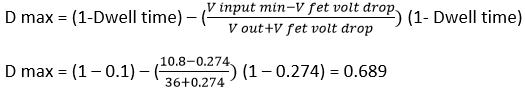Find the minimum duty ratio, D min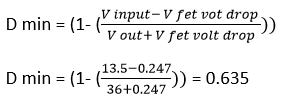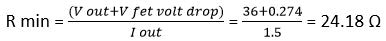Find the maximum inductance, L max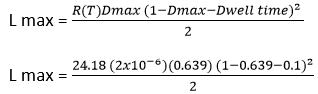L max = 0.74 uHy

Find the peak current, I pk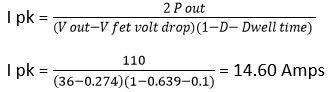Assuming the efficiency is 93%, I pk = 15.7 Amps.

Find the RMS current, I rms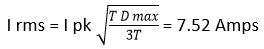From this point on you can finish the design or use TT Electronics (TTE) Inductor Datasheet for choosing an optimum inductance and size required.  Lower/higher inductance can be chosen and the current ratings will be close to the calculation but the performance should be verified using the test bench.

For this example, a good suggestion is TTE p/n: HA72L-06241R5LFTR for the automotive grade, -55 ºC to +155 ºC (AEC-Q200 Certified) and has following electrical parameters:

Table 1. Electrical specs (Transferred from the datasheet).

TTE p/n L @ 0 Amp +/- 20% DCR typical DCR max I rms Temp rise to 40 ºC I dc (sat) 30% Roll off Typical picture
HA72L-06241R0LFTR 1.00 13.1 14.2 9.0 16.0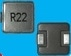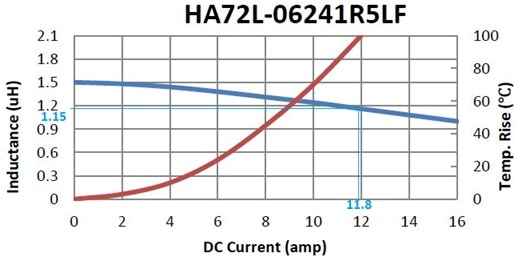Figure 2. DC bias and Temperature rise curve.

Figure 2. shows the inductance roll off is about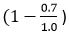100 = 30% at 15.7 Amps. Since the calculated RMS current is 7.52 A, this is well below 9.0 A shown in table 1 above. So, the expected temperature rise* would be far less than 40 ºC. All off the performances must be tested on the test bench as usual.

* In this example, Irms was given by the inductor manufacturer that can be employed as a reference point (40 ºC rise) to establish the temperature rise. The temperature rise would be above, below or equal to the reference point. For an accurate temperature rise calculation, we must know the following parameters, but not limited to:

1. Ohms/1000 feet of the wire used (your preference of unit measurement)
2. Total number of turns
3. Mean Length Turn information
4. Bac (ac flux density) intended to use (depends on the core material)
1. It can be calculated if gap is known (this case Magnetics Path Length)
5. Skin depth information
6. Copper loss can be calculated from the information stated above
7. Core loss; milliwatts/gram or watt/Lbs.
8. Surface Area of the inductor (can be calculated)

TT Electronics has provided numerous solutions to the medical, automotive, and various industrial sectors. The company provides semi and full custom designs to meet your critical specifications.This information has been sourced, reviewed and adapted from materials provided by TT Electronics.

## Citations

• APA

TT Electronics plc. (2019, October 29). A Guide to Choosing a Proper Inductor for DC-DC Boost Applications. AZoSensors. Retrieved on February 24, 2020 from https://www.azosensors.com/article.aspx?ArticleID=1645.

• MLA

TT Electronics plc. "A Guide to Choosing a Proper Inductor for DC-DC Boost Applications". AZoSensors. 24 February 2020. <https://www.azosensors.com/article.aspx?ArticleID=1645>.

• Chicago

TT Electronics plc. "A Guide to Choosing a Proper Inductor for DC-DC Boost Applications". AZoSensors. https://www.azosensors.com/article.aspx?ArticleID=1645. (accessed February 24, 2020).

• Harvard

TT Electronics plc. 2019. A Guide to Choosing a Proper Inductor for DC-DC Boost Applications. AZoSensors, viewed 24 February 2020, https://www.azosensors.com/article.aspx?ArticleID=1645.

## Tell Us What You Think